# Lecture 3 OUTLINE Semiconductor Basics contd Carrier drift

• Slides: 22Lecture 3 OUTLINE • Semiconductor Basics (cont’d) – Carrier drift and diffusion • PN Junction Diodes – Electrostatics – Capacitance Reading: Chapter 2. 1 -2. 2 EE 105 Fall 2011 Lecture 3, Slide 1 Prof. Salahuddin, UC BerkeleyRecap: Drift Current • Drift current is proportional to the carrier velocity and carrier concentration: Total current Jp, drift= Q/t Q= total charge contained in the volume shown to the right t= time taken by Q to cross the volume Q=qp(in cm 3)X Volume=qp. AL=qp. Avht Hole current per unit area (i. e. current density) Jp, drift = q p vh EE 105 Fall 2011 Lecture 3, Slide 2 Prof. Salahuddin, UC Berkeley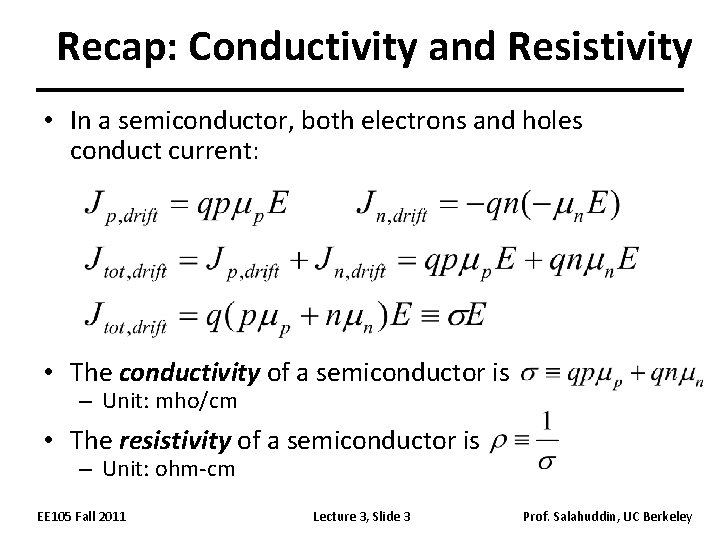Recap: Conductivity and Resistivity • In a semiconductor, both electrons and holes conduct current: • The conductivity of a semiconductor is – Unit: mho/cm • The resistivity of a semiconductor is – Unit: ohm-cm EE 105 Fall 2011 Lecture 3, Slide 3 Prof. Salahuddin, UC BerkeleyElectrical Resistance I V + _ W t homogeneously doped sample L (Unit: ohms) Resistance where r is the resistivity EE 105 Fall 2011 Lecture 3, Slide 4 Prof. Salahuddin, UC Berkeley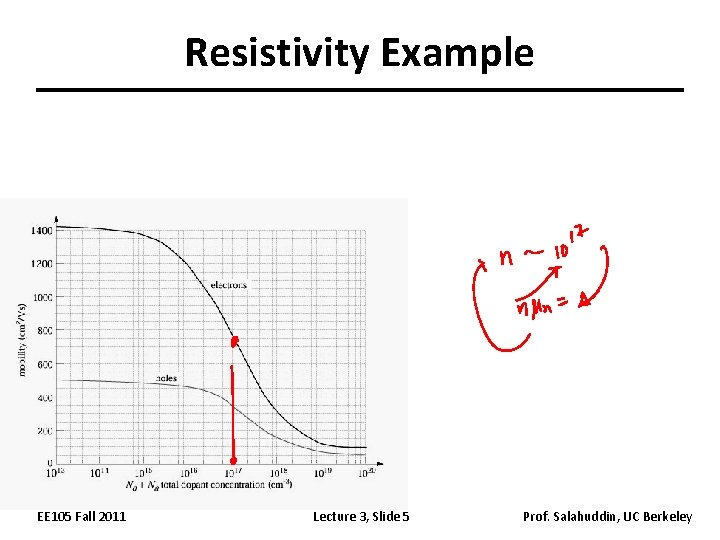Resistivity Example EE 105 Fall 2011 Lecture 3, Slide 5 Prof. Salahuddin, UC BerkeleyA Second Mechanism of Current Flow is Diffusion EE 105 Fall 2011 Lecture 3, Slide 6 Prof. Salahuddin, UC BerkeleyCarrier Diffusion • Due to thermally induced random motion, mobile particles tend to move from a region of high concentration to a region of low concentration. – Analogy: ink droplet in water EE 105 Fall 2011 Lecture 3, Slide 7 Prof. Salahuddin, UC BerkeleyCarrier Diffusion • Current flow due to mobile charge diffusion is proportional to the carrier concentration gradient. – The proportionality constant is the diffusion constant. Notation: Dp hole diffusion constant (cm 2/s) Dn electron diffusion constant (cm 2/s) EE 105 Fall 2011 Lecture 3, Slide 8 Prof. Salahuddin, UC Berkeley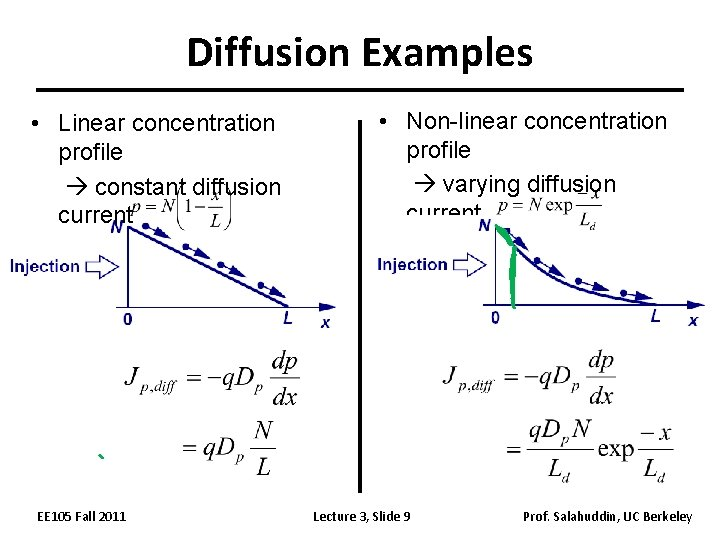Diffusion Examples • Linear concentration profile constant diffusion current EE 105 Fall 2011 • Non-linear concentration profile varying diffusion current Lecture 3, Slide 9 Prof. Salahuddin, UC Berkeley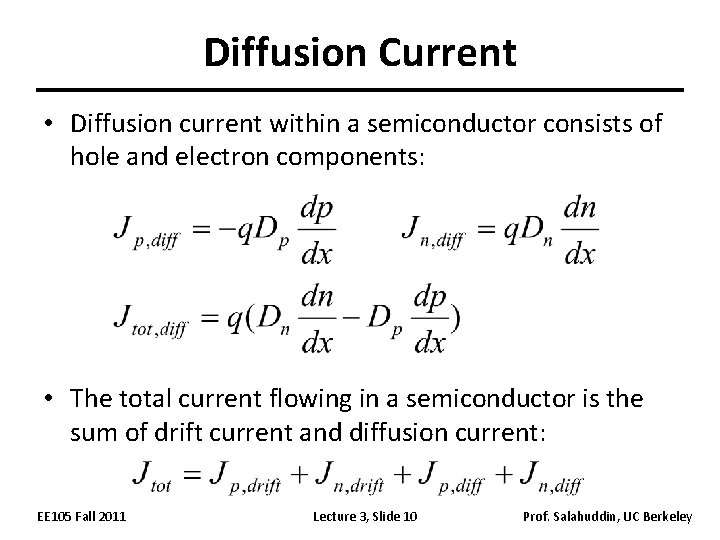Diffusion Current • Diffusion current within a semiconductor consists of hole and electron components: • The total current flowing in a semiconductor is the sum of drift current and diffusion current: EE 105 Fall 2011 Lecture 3, Slide 10 Prof. Salahuddin, UC Berkeley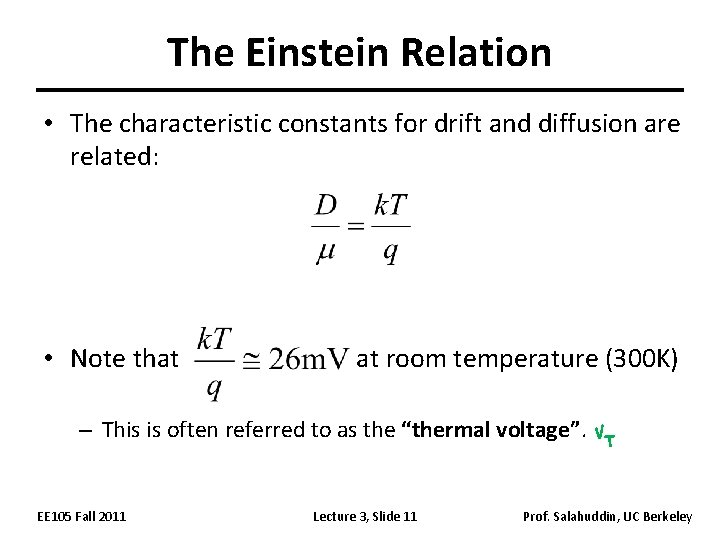The Einstein Relation • The characteristic constants for drift and diffusion are related: • Note that at room temperature (300 K) – This is often referred to as the “thermal voltage”. EE 105 Fall 2011 Lecture 3, Slide 11 Prof. Salahuddin, UC BerkeleyThe PN Junction Diode • When a P-type semiconductor region and an N-type semiconductor region are in contact, a PN junction diode is formed. V – D + ID EE 105 Fall 2011 Lecture 3, Slide 12 Prof. Salahuddin, UC Berkeley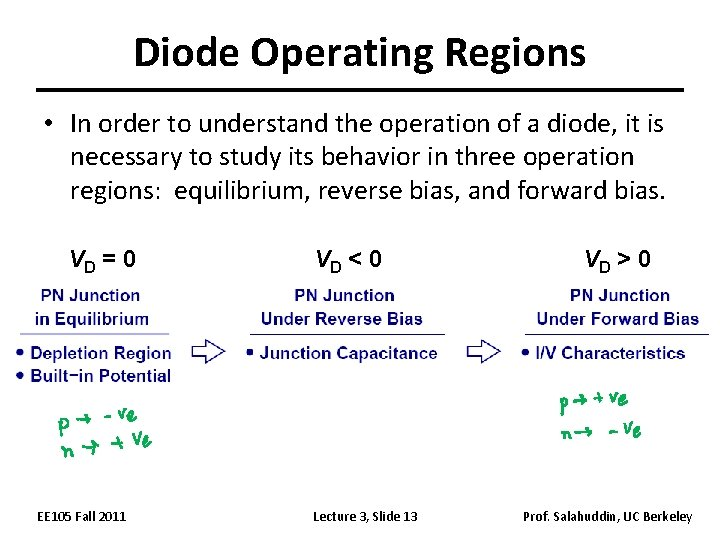Diode Operating Regions • In order to understand the operation of a diode, it is necessary to study its behavior in three operation regions: equilibrium, reverse bias, and forward bias. VD = 0 EE 105 Fall 2011 VD < 0 Lecture 3, Slide 13 VD > 0 Prof. Salahuddin, UC Berkeley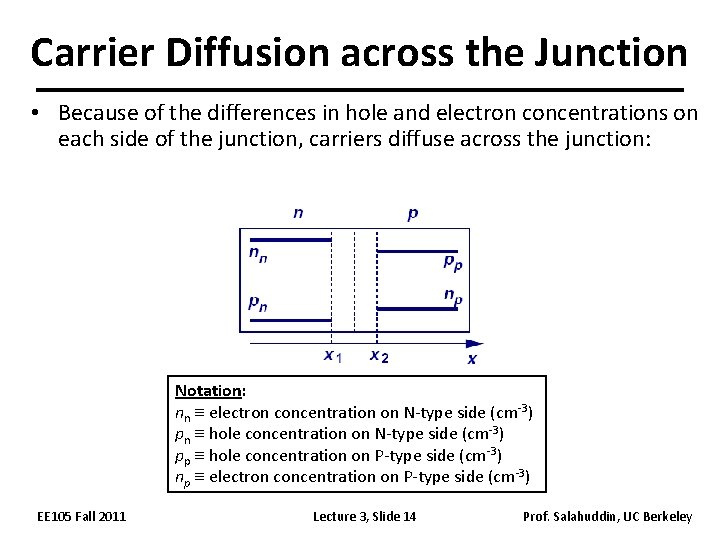Carrier Diffusion across the Junction • Because of the differences in hole and electron concentrations on each side of the junction, carriers diffuse across the junction: Notation: nn electron concentration on N-type side (cm-3) pn hole concentration on N-type side (cm-3) pp hole concentration on P-type side (cm-3) np electron concentration on P-type side (cm-3) EE 105 Fall 2011 Lecture 3, Slide 14 Prof. Salahuddin, UC BerkeleyDepletion Region • As conduction electrons and holes diffuse across the junction, they leave behind ionized dopants. Thus, a region that is depleted of mobile carriers is formed. – The charge density in the depletion region is not zero. – The carriers which diffuse across the junction recombine with majority carriers, i. e. they are annihilated. quasineutral region width=Wdep region EE 105 Fall 2011 Lecture 3, Slide 15 Prof. Salahuddin, UC Berkeley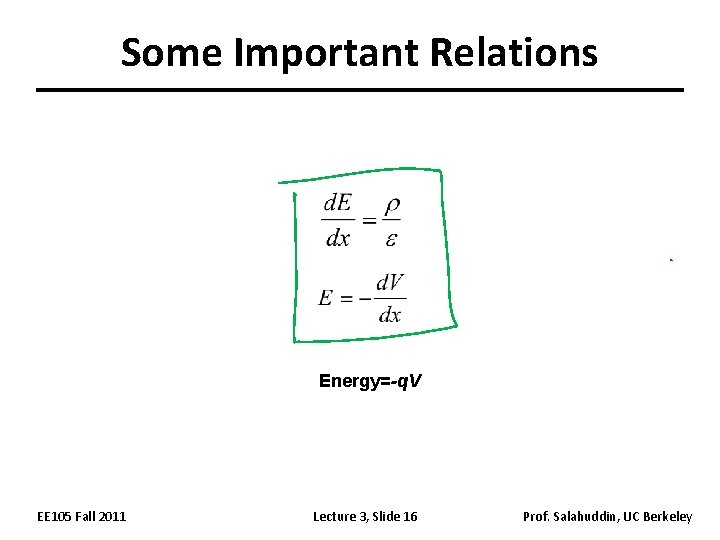Some Important Relations Energy=-q. V EE 105 Fall 2011 Lecture 3, Slide 16 Prof. Salahuddin, UC BerkeleyThe Depletion Approximation Because charge density ≠ 0 in the depletion region, a large E-field exists in this region: In the depletion region on the N side: r(x) In the depletion region on the P side: q. ND a -b -q. NA EE 105 Fall 2011 x Lecture 3, Slide 17 Prof. Salahuddin, UC Berkeley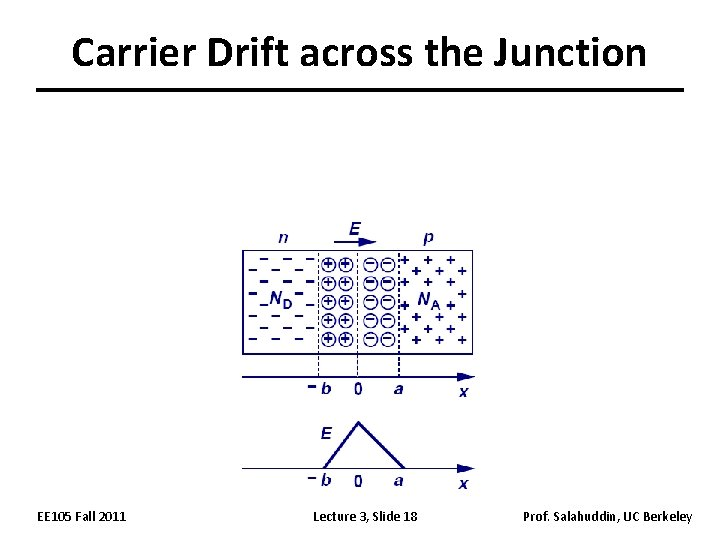Carrier Drift across the Junction EE 105 Fall 2011 Lecture 3, Slide 18 Prof. Salahuddin, UC Berkeley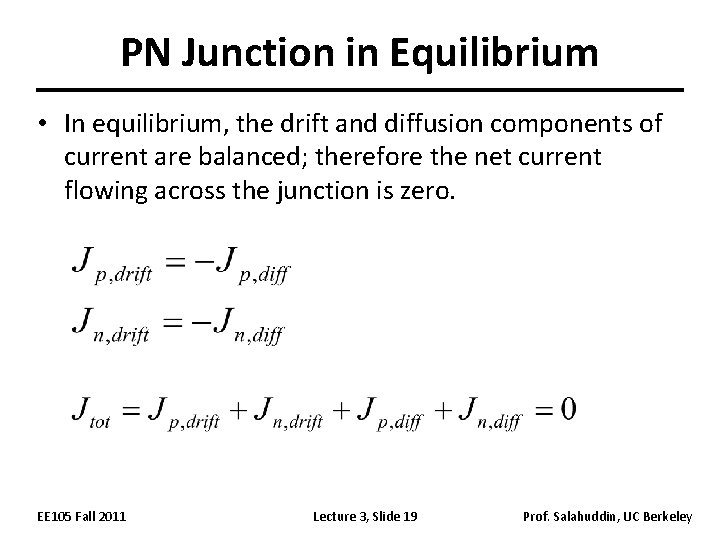PN Junction in Equilibrium • In equilibrium, the drift and diffusion components of current are balanced; therefore the net current flowing across the junction is zero. EE 105 Fall 2011 Lecture 3, Slide 19 Prof. Salahuddin, UC Berkeley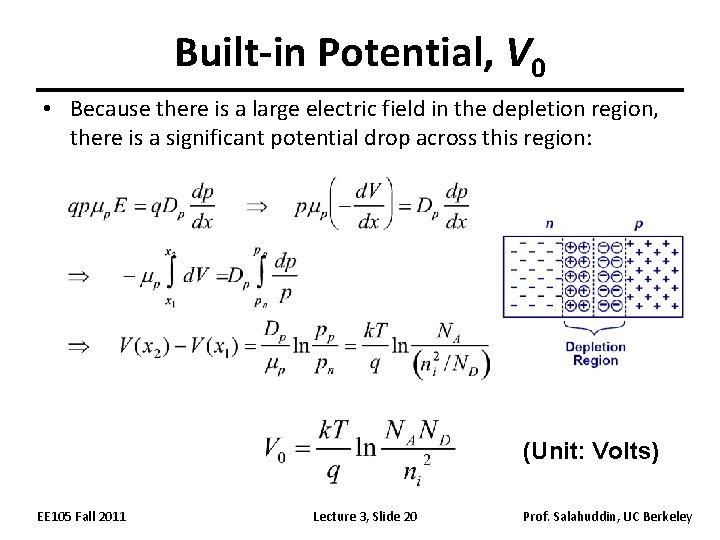Built-in Potential, V 0 • Because there is a large electric field in the depletion region, there is a significant potential drop across this region: (Unit: Volts) EE 105 Fall 2011 Lecture 3, Slide 20 Prof. Salahuddin, UC Berkeley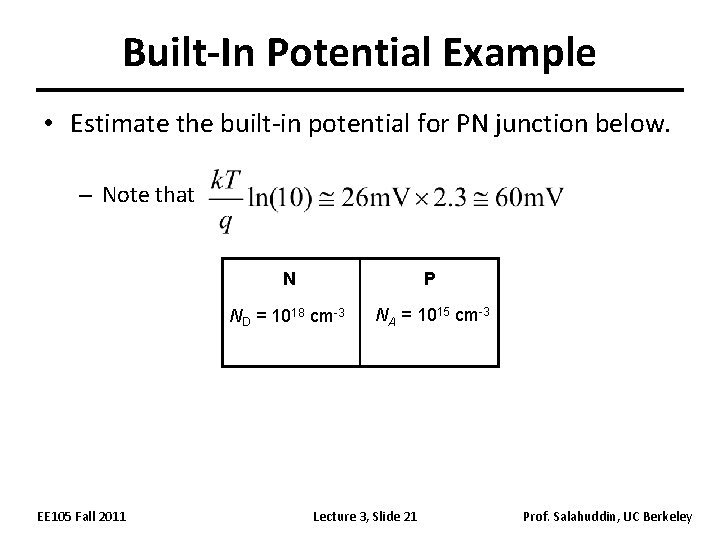Built-In Potential Example • Estimate the built-in potential for PN junction below. – Note that EE 105 Fall 2011 N P ND = 1018 cm-3 NA = 1015 cm-3 Lecture 3, Slide 21 Prof. Salahuddin, UC Berkeley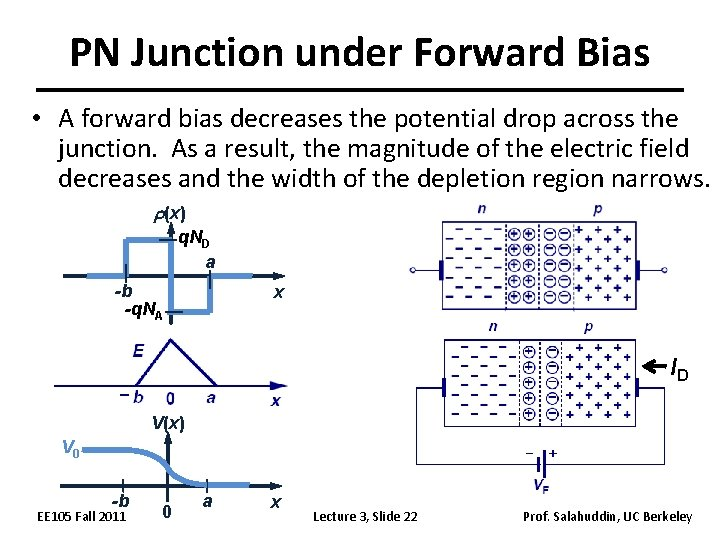PN Junction under Forward Bias • A forward bias decreases the potential drop across the junction. As a result, the magnitude of the electric field decreases and the width of the depletion region narrows. r(x) q. ND a -b -q. NA x ID V(x) V 0 -b EE 105 Fall 2011 0 a x Lecture 3, Slide 22 Prof. Salahuddin, UC Berkeley